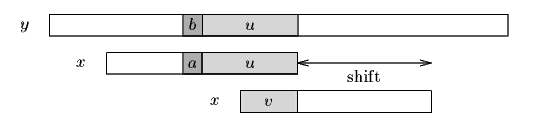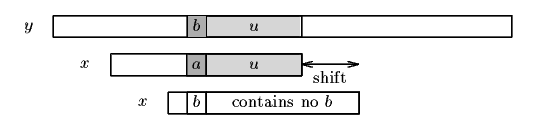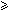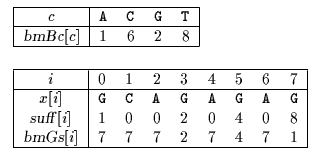Next: Turbo-BM algorithm Up: ESMAJ Prev: Not So Naive algorithm

# Boyer-Moore algorithm

Main features
• performs the comparisons from right to left;
• preprocessing phase in O(m+) time and space complexity;
• searching phase in O(mn) time complexity;
• 3n text character comparisons in the worst case when searching for a non periodic pattern;
• O(n / m) best performance.
Description

The Boyer-Moore algorithm is considered as the most efficient string-matching algorithm in usual applications. A simplified version of it or the entire algorithm is often implemented in text editors for the «search» and «substitute» commands.

The algorithm scans the characters of the pattern from right to left beginning with the rightmost one. In case of a mismatch (or a complete match of the whole pattern) it uses two precomputed functions to shift the window to the right. These two shift functions are called the good-suffix shift (also called matching shift and the bad-character shift (also called the occurrence shift).

Assume that a mismatch occurs between the character x[i]=a of the pattern and the character y[i+j]=b of the text during an attempt at position j.
Then, x[i+1 .. m-1]=y[i+j+1 .. j+m-1]=u and x[iy[i+j]. The good-suffix shift consists in aligning the segment y[i+j+1 .. j+m-1]=x[i+1 .. m-1] with its rightmost occurrence in x that is preceded by a character different from x[i] (see figure 13.1).Figure 13.1. The good-suffix shift, u re-occurs preceded by a character c different from a.

If there exists no such segment, the shift consists in aligning the longest suffix v of y[i+j+1 .. j+m-1] with a matching prefix of x (see figure 13.2).Figure 13.2. The good-suffix shift, only a suffix of u re-occurs in x.

The bad-character shift consists in aligning the text character y[i+j] with its rightmost occurrence in x[0 .. m-2]. (see figure 13.3)Figure 13.3. The bad-character shift, a occurs in x.

If y[i+j] does not occur in the pattern x, no occurrence of x in y can include y[i+j], and the left end of the window is aligned with the character immediately after y[i+j], namely y[i+j+1] (see figure 13.4).Figure 13.4. The bad-character shift, b does not occur in x.

Note that the bad-character shift can be negative, thus for shifting the window, the Boyer-Moore algorithm applies the maximum between the the good-suffix shift and bad-character shift. More formally the two shift functions are defined as follows.

The good-suffix shift function is stored in a table bmGs of size m+1.

Let us define two conditions:Cs(i, s): for each k such that i < k < m, sk or x[k-s]=x[k] andCo(i, s): if s

Then, fori < m: bmGs[i+1]=min{s>0 : Cs(i, s) and Co(i, s) hold}
and we define bmGs as the length of the period of x. The computation of the table bmGs use a table suff defined as follows: for 1i < m, suff[i]=max{k : x[i-k+1 .. i]=x[m-k .. m-1]}

The bad-character shift function is stored in a table bmBc of size. For c in: bmBc[c] = min{i : 1i <m-1 and x[m-1-i]=c} if c occurs in x, m otherwise.

Tables bmBc and bmGs can be precomputed in time O(m+) before the searching phase and require an extra-space in O(m+). The searching phase time complexity is quadratic but at most 3n text character comparisons are performed when searching for a non periodic pattern. On large alphabets (relatively to the length of the pattern) the algorithm is extremely fast. When searching for am-1b in bn the algorithm makes only O(n / m) comparisons, which is the absolute minimum for any string-matching algorithm in the model where the pattern only is preprocessed.

The C code
```void preBmBc(char *x, int m, int bmBc[]) {
int i;

for (i = 0; i < ASIZE; ++i)
bmBc[i] = m;
for (i = 0; i < m - 1; ++i)
bmBc[x[i]] = m - i - 1;
}

void suffixes(char *x, int m, int *suff) {
int f, g, i;

suff[m - 1] = m;
g = m - 1;
for (i = m - 2; i >= 0; --i) {
if (i > g && suff[i + m - 1 - f] < i - g)
suff[i] = suff[i + m - 1 - f];
else {
if (i < g)
g = i;
f = i;
while (g >= 0 && x[g] == x[g + m - 1 - f])
--g;
suff[i] = f - g;
}
}
}

void preBmGs(char *x, int m, int bmGs[]) {
int i, j, suff[XSIZE];

suffixes(x, m, suff);

for (i = 0; i < m; ++i)
bmGs[i] = m;
j = 0;
for (i = m - 1; i >= 0; --i)
if (suff[i] == i + 1)
for (; j < m - 1 - i; ++j)
if (bmGs[j] == m)
bmGs[j] = m - 1 - i;
for (i = 0; i <= m - 2; ++i)
bmGs[m - 1 - suff[i]] = m - 1 - i;
}

void BM(char *x, int m, char *y, int n) {
int i, j, bmGs[XSIZE], bmBc[ASIZE];

/* Preprocessing */
preBmGs(x, m, bmGs);
preBmBc(x, m, bmBc);

/* Searching */
j = 0;
while (j <= n - m) {
for (i = m - 1; i >= 0 && x[i] == y[i + j]; --i);
if (i < 0) {
OUTPUT(j);
j += bmGs;
}
else
j += MAX(bmGs[i], bmBc[y[i + j]] - m + 1 + i);
}
}

```
The example

Preprocessing phasebmBc and bmGs tables used by Boyer-Moore algorithm

Searching phase

References
• AHO, A.V., 1990, Algorithms for finding patterns in strings. in Handbook of Theoretical Computer Science, Volume A, Algorithms and complexity, J. van Leeuwen ed., Chapter 5, pp 255-300, Elsevier, Amsterdam.
• AOE, J.-I., 1994, Computer algorithms: string pattern matching strategies, IEEE Computer Society Press.
• BAASE, S., VAN GELDER, A., 1999, Computer Algorithms: Introduction to Design and Analysis, 3rd Edition, Chapter 11, pp. ??-??, Addison-Wesley Publishing Company.
• BAEZA-YATES R., NAVARRO G., RIBEIRO-NETO B., 1999, Indexing and Searching, in Modern Information Retrieval, Chapter 8, pp 191-228, Addison-Wesley.
• BEAUQUIER, D., BERSTEL, J., CHRÉTIENNE, P., 1992, Éléments d'algorithmique, Chapter 10, pp 337-377, Masson, Paris.
• BOYER R.S., MOORE J.S., 1977, A fast string searching algorithm. Communications of the ACM. 20:762-772.
• COLE, R., 1994, Tight bounds on the complexity of the Boyer-Moore pattern matching algorithm, SIAM Journal on Computing 23(5):1075-1091.
• CORMEN, T.H., LEISERSON, C.E., RIVEST, R.L., 1990. Introduction to Algorithms, Chapter 34, pp 853-885, MIT Press.
• CROCHEMORE, M., 1997. Off-line serial exact string searching, in Pattern Matching Algorithms, ed. A. Apostolico and Z. Galil, Chapter 1, pp 1-53, Oxford University Press.
• CROCHEMORE, M., HANCART, C., 1999, Pattern Matching in Strings, in Algorithms and Theory of Computation Handbook, M.J. Atallah ed., Chapter 11, pp 11-1--11-28, CRC Press Inc., Boca Raton, FL.
• CROCHEMORE, M., LECROQ, T., 1996, Pattern matching and text compression algorithms, in CRC Computer Science and Engineering Handbook, A. Tucker ed., Chapter 8, pp 162-202, CRC Press Inc., Boca Raton, FL.
• CROCHEMORE, M., RYTTER, W., 1994, Text Algorithms, Oxford University Press.
• GONNET, G.H., BAEZA-YATES, R.A., 1991. Handbook of Algorithms and Data Structures in Pascal and C, 2nd Edition, Chapter 7, pp. 251-288, Addison-Wesley Publishing Company.
• GOODRICH, M.T., TAMASSIA, R., 1998, Data Structures and Algorithms in JAVA, Chapter 11, pp 441-467, John Wiley & Sons.
• GUSFIELD, D., 1997, Algorithms on strings, trees, and sequences: Computer Science and Computational Biology, Cambridge University Press.
• HANCART, C., 1993. Analyse exacte et en moyenne d'algorithmes de recherche d'un motif dans un texte, Ph. D. Thesis, University Paris 7, France.
• KNUTH, D.E., MORRIS (Jr) J.H., PRATT, V.R., 1977, Fast pattern matching in strings, SIAM Journal on Computing 6(1):323-350.
• LECROQ, T., 1992, Recherches de mot, Ph. D. Thesis, University of Orléans, France.
• LECROQ, T., 1995, Experimental results on string matching algorithms, Software - Practice & Experience 25(7):727-765.
• SEDGEWICK, R., 1988, Algorithms, Chapter 19, pp. 277-292, Addison-Wesley Publishing Company.
• SEDGEWICK, R., 1988, Algorithms in C, Chapter 19, Addison-Wesley Publishing Company.
• STEPHEN, G.A., 1994, String Searching Algorithms, World Scientific.
• WATSON, B.W., 1995, Taxonomies and Toolkits of Regular Language Algorithms, Ph. D. Thesis, Eindhoven University of Technology, The Netherlands.
• WIRTH, N., 1986, Algorithms & Data Structures, Chapter 1, pp. 17-72, Prentice-Hall.Next: Turbo-BM algorithm Up: ESMAJ Prev: Not So Naive algorithm

e-mails: {Christian.Charras, Thierry.Lecroq }@laposte.net
Tue Jan 14 15:03:31 MET 1997